# Selina solutions for Concise Mathematics Class 7 ICSE chapter 16 - Pythagoras Theorem [Latest edition]

#### Chapters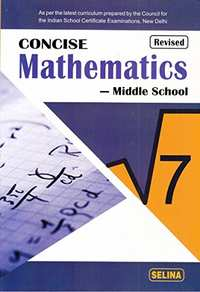## Chapter 16: Pythagoras Theorem

Exercise 16
Exercise 16

### Selina solutions for Concise Mathematics Class 7 ICSE Chapter 16 Pythagoras TheoremExercise 16

Exercise 16 | Q 1

Triangle ABC is right-angled at vertex A. Calculate the length of BC, if AB = 18 cm and AC = 24 cm.

Exercise 16 | Q 2

Triangle XYZ is right-angled at vertex Z. Calculate the length of YZ, if XY = 13 cm and XZ = 12 cm.

Exercise 16 | Q 3

Triangle PQR is right-angled at vertex R. Calculate the length of PR, if: PQ = 34 cm and QR = 33.6 cm.

Exercise 16 | Q 4.1

The sides of a certain triangle is given below. Find, which of them is right-triangle

16 cm, 20 cm, and 12 cm

Exercise 16 | Q 4.2

The sides of a certain triangle is given below. Find, which of them is right-triangle

6 m, 9 m, and 13 m

Exercise 16 | Q 5

In the given figure, angle BAC = 90°, AC = 400 m, and AB = 300 m. Find the length of BC.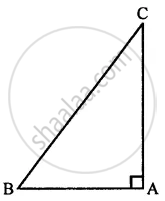Exercise 16 | Q 6

In the given figure, angle ACP = ∠BDP = 90°, AC = 12 m, BD = 9 m and PA= PB = 15 m. Find:
(i) CP
(ii) PD
(iii) CD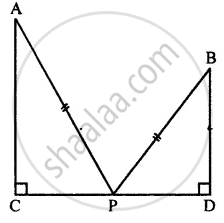Exercise 16 | Q 7.1

In triangle PQR, angle Q = 90°, find: PR, if PQ = 8 cm and QR = 6 cm

Exercise 16 | Q 7.2

In triangle PQR, angle Q = 90°, find: PQ, if PR = 34 cm and QR = 30 cm

Exercise 16 | Q 8

Show that the triangle ABC is a right-angled triangle; if: AB = 9 cm, BC = 40 cm and AC = 41 cm

Exercise 16 | Q 9

In the given figure, angle ACB = 90° = angle ACD. If AB = 10 m, BC = 6 cm and AD = 17 cm, find :
(i) AC
(ii) CD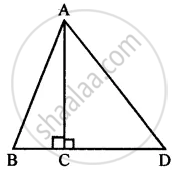Exercise 16 | Q 10

In the given figure, angle ADB = 90°, AC = AB = 26 cm and BD = DC. If the length of AD = 24 cm; find the length of BC.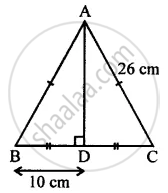Exercise 16 | Q 11

In the given figure, AD = 13 cm, BC = 12 cm, AB = 3 cm and angle ACD = angle ABC = 90°. Find the length of DC.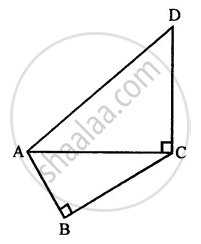Exercise 16 | Q 12

A ladder, 6.5 m long, rests against a vertical wall. If the foot of the ladder is 2.5 m from the foot of the wall, find up to how much height does the ladder reach?

Exercise 16 | Q 13

A boy first goes 5 m due north and then 12 m due east. Find the distance between the initial and the final position of the boy.

Exercise 16 | Q 14

Use the information given in the figure to find the length AD.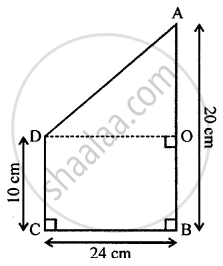## Chapter 16: Pythagoras Theorem

Exercise 16## Selina solutions for Concise Mathematics Class 7 ICSE chapter 16 - Pythagoras Theorem

Selina solutions for Concise Mathematics Class 7 ICSE chapter 16 (Pythagoras Theorem) include all questions with solution and detail explanation. This will clear students doubts about any question and improve application skills while preparing for board exams. The detailed, step-by-step solutions will help you understand the concepts better and clear your confusions, if any. Shaalaa.com has the CISCE Concise Mathematics Class 7 ICSE solutions in a manner that help students grasp basic concepts better and faster.

Further, we at Shaalaa.com provide such solutions so that students can prepare for written exams. Selina textbook solutions can be a core help for self-study and acts as a perfect self-help guidance for students.

Concepts covered in Concise Mathematics Class 7 ICSE chapter 16 Pythagoras Theorem are Right-angled Triangles and Pythagoras Property.

Using Selina Class 7 solutions Pythagoras Theorem exercise by students are an easy way to prepare for the exams, as they involve solutions arranged chapter-wise also page wise. The questions involved in Selina Solutions are important questions that can be asked in the final exam. Maximum students of CISCE Class 7 prefer Selina Textbook Solutions to score more in exam.

Get the free view of chapter 16 Pythagoras Theorem Class 7 extra questions for Concise Mathematics Class 7 ICSE and can use Shaalaa.com to keep it handy for your exam preparation